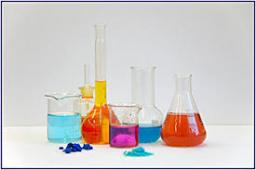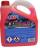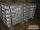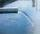# Coolant

The driver of the car cooler filled with a mixture of 3.9 liters and 2.6 liters of water antifreeze coolant. At what rate are this two components of the mixture?

Correct result:

p =  1.5

#### Solution:

$p=3.9/2.6=\dfrac{ 3 }{ 2 }=1.5$We would be pleased if you find an error in the word problem, spelling mistakes, or inaccuracies and send it to us. Thank you!Tips to related online calculators
Check out our ratio calculator.
Tip: Our volume units converter will help you with the conversion of volume units.

## Next similar math problems:

• Cork and swimmingIf a person weighs 80 kg, how many kilograms of cork must take swimming belt to use it to float on water? The density of the human body is 1050kg/m3 and cork 300kg/m3. (Instructions: Let the human body and cork on a mixture that has a density of 1000kg/m3
• SolutionWhat amount of 80% solution and what amount of water is needed to mix in order to obtain two liters of 60% solution of this mixture?
• Cooling liquidCooling liquid is diluted with water in a ratio of 3:2 (3 parts by volume of coolant with 2 volumes of water). How many volumes of coolant must be prepared for a total 0.7 dm3 (liters) of the mixture?
• AlloyThe first alloy is a mixture of two metals in the ratio 1:2, the second is a mixture of same metals in the ratio 2:3. At what ratio we have these two alloys put into the furnace to obtain a new metal alloy with ratio 17:27? (All three ratios correspond to
• Mixture of nutsThe mixture of nuts should be prepared from almonds, peanuts and cashew nuts ratio 1: 2: 3 (respectively). The price of almonds is 150 CZK/kg, the price of peanuts is 140 CZK/kg and the price of cashew nuts is 180 CZK/kg. The price of the mixture is deter
• RainfallA rectangular garden of 25m in length and width 20m in width fall 4mm of water. Express by a fraction in basic form what part of the 60-hectolitre tank we would fill with this water.
• Water tankA 288 hectoliter of water was poured into the tank with dimensions 12 m and 6 m bottom and 2 m depth. What part of the volume of the tank water occupied? Calculate the surface of tank wetted with water.
• Water inletsInlet valve with a flow rate of 12 liters per second is filled tank for 72 minutes. How long take to fill full tank if we open one more such valve half an hour after?
• Mixture 2How many liters of water must be added to 7 liters of a 20% solution to obtain a 10% solution?
• SugarsIn what ratio must two sorts of sugar, costing #390 and #315 per kg respectively, be mixed in order to produce a mixture worth #369 per kg?
• Alcohol 2Two types of alcohol one 63% and second 75% give 20 liters of 69% alcohol. How many liters of each type are in the mixture?
• Vinegar 2How many percentage of getvinegar solution, if we mix to 3.5 liters of 5.8% and 5 liters of 7.6% vinegar?
• Tea blendTea blends are maked from two kinds of tea. In standard tea mixture are two teas in the ratio 1:3 and 40 g costs 42 CZK. In the premium tea mixture are weighing two teas in the ratio 1:1 and 50 grams costs 60 CZK. How much cost 10 grams of more expensive
• CoffeeIn stock are three kinds of branded coffee prices: I. kind......205 Kc/kg II. kind......274 Kc/kg III. kind.....168 Kc/kg Mixing these three species in the ratio 8:5:6 create a mixture. What will be the price of 100 grams of this mixture?
• Mixing paint with waterMr. Adamek will paint. The purchased paint is diluted with water in a ratio of 1: 1.5. a) how many parts of water will add to 1 part of the paint b) how many liters of water the mission adds to 2 liters of paint
• Sea waterSeawater contains about 4.3% salt. How many dm3 of distilled water we must pour into 5 dm3 of sea water to get water with 1.8% salt?
• ExcavationMr. Billy calculated that excavation for a water connection dig for 12 days. His friend would take 10 days. Billy worked 3 days alone. Then his friend came to help and started on the other end. On what day since the beginning of excavation they met?